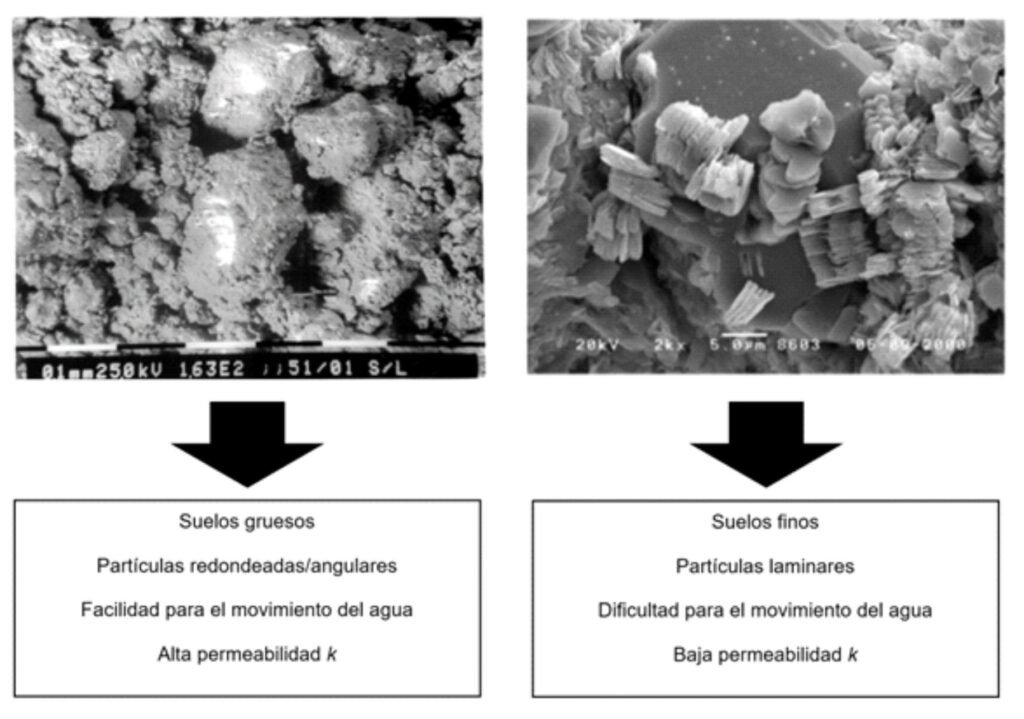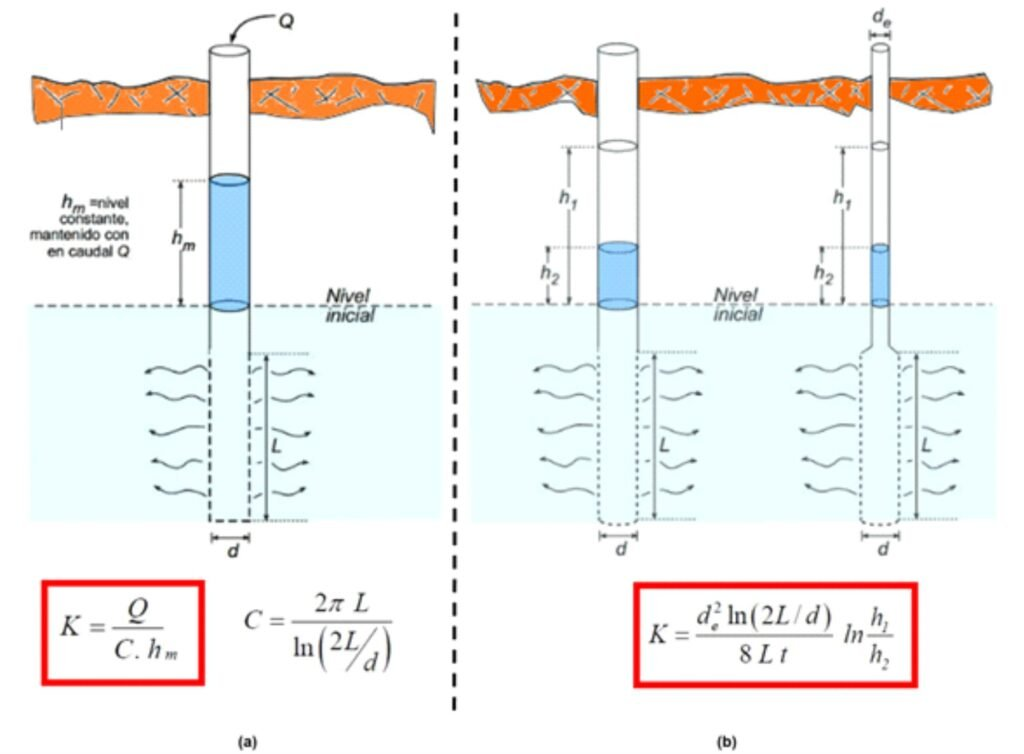## "If you can't explain it simply, you don't understand it well enough" (Albert Einstein)## Measurement of the in situ permeability coefficient for soils: THE LEFRANC METHOD

The permeability coefficient is a fundamental parameter for the analysis of groundwater flow in soils. However, its determination in the laboratory has limitations, particularly in the case of granular soils, where it is not possible to obtain undisturbed samples. Therefore, the best approach to estimate the permeability coefficient of soils is through in situ tests. Do you want to learn more about this topic? Continue reading this post...

### Factors affecting soil permeability

Permeability is the capacity of certain material to allow a fluid to pass through it without altering its composition. In Soil Mechanics, the parameter used to quantify this property is the permeability coefficient "k", which has units of velocity and represents, from the physical point of view, the greater or lesser ease with which water can move through the soil grains. Thus, a relatively high value of k implies that permeability is high (as is the case of granular soils), while lower values of k imply lower permeability (typical of fine soils).

Among the different factors that influence the permeability of a given soil, it is important to mention the following:

1. a) Grain size, since soil voids are usually proportional to the size of its grains.
2. b) Fluid properties, especially temperature
3. c) The void ratio of the soil, since water moves in the soil through its pores
4. d) The shape and arrangement of the grains.
5. e) The degree of saturation.
6. f) The chemical properties of the soil, fundamentally for the case of clays and rocks

As can be seen from the above list, all of these factors are directly related to the in situ soil conditions, specifically the arrangement of the grains in the soil. This can be seen in a simple way in Figure 1.In lab, it is possible to estimate the permeability coefficient k of predominantly coarse materials by means of the constant load permeability test, and of finer materials by means of the variable load permeability test. However, the main limitation associated with these tests is that the samples are not always representative of the arrangement of soil grains in the field. This is particularly true for granular soils, which implies that the values of the permeability coefficient k obtained in the laboratory usually represent an order of magnitude larger than the in situ permeability value.

En laboratorio es posible estimar el coeficiente de permeabilidad k de materiales predominantemente gruesos por medio del ensayo de permeabilidad a carga constante, y de materiales más finos a través del ensayo de permeabilidad de carga variable. Sin embargo, la principal limitación asociada a estos ensayos es que las muestras no son siempre representativas del arreglo que presentan los granos de suelo en campo. Esto resulta particularmente cierto para el caso de suelos granulares, lo cual implica que los valores del coeficiente de permeabilidad k obtenidos en laboratorio usualmente representen un orden de magnitud grueso del valor de la permeabilidad in situ.

As a result, in situ permeability tests yield results that are closer to the reality of the ground, mainly because they are not influenced by the extraction, transport, and preparation of the sample, and, in addition, the scale of the test is adjusted to the ground.

### In-situ permeability in soils: the Lefranc test

Numerous methods for measuring the permeability of soils in situ have been proposed over the years. The Lefranc method, originally developed around 1936, is one of the most widely used in practice, mainly because of its relatively simple execution.

Este ensayo se utiliza para medir el coeficiente de permeabilidad en suelos permeables o semi-permeables (es decir, en materiales predominantemente granulares) situados por debajo del nivel freático; y puede emplearse también en rocas muy fracturadas.

The Lefranc test is governed by ASTM D-2573, and has two variables, as shown in Figure 2: A) the constant regime test (corresponding to part "a" of the figure); and B) the variable regime test (corresponding to part "b"). The expressions used in each case for the determination of the coefficient k are shown in the same plate. k.Figure 2 The Lefranc test (Source: modified from https://geologiaweb.com/ingenieria-geologica/ensayo-lefranc/).

A) The Lefranc test at constant regime

In the case of the constant regime (see part "a" of Figure 2), the procedure consists of filling a borehole with water (typically a borehole is used after all the samples have been taken during the field exploration process) and measuring the flow rate Q necessary to maintain the constant level.

This flow rate is measured every 5 minutes, and the borehole level must be kept constant for 45 minutes. If there is a high permeability soil, it will be necessary to introduce a lot of water into the borehole, and in that case the flow rate should be measured every 1 minute for the first 20 minutes of the test, and then every 5 minutes.

Once the measurements have been made, the permeability coefficient k is obtained with the expression shown in part "a" of Figure 2, taking into account the average value of all the flow Q measurements made. The coefficient C included in this expression is associated with the shape of the borehole, and depends on purely geometrical parameters, such as the length and diameter of the borehole.

B) The Lefranc test at variable regime

The variable regime Lefranc test (see part "b" of Figure 2) involves the measurement of the rate of descent of the water level inside the borehole. In this case, the water load must not exceed 10 m measured from the center of the filter chamber, i.e. from the center of the borehole section with casing.

During the test, at least 5 measurements must be made of the following parameters: h1, which is the initial water level inside the borehole; and h2, which is the water level to which it descends after a time t, to be measured from the beginning of the test.

Knowing these parameters, it is possible to determine the permeability k using the expression shown in part "b" of Figure 2.

### The accuracy of the Lefranc test

One question that is very important to know the answer to is: the permeability of which stratum is being measured when performing the Lefranc test, either the steady-state or the variable-state test?

As shown in Figure 2, the test length L (i.e., the thickness of the stratum for which the permeability is being measured) is defined between the end of the borehole casing (which is necessary so that the soil does not collapse) and the bottom of the borehole. That is the stratum for which the determined k value is valid.

However, it is important to note that different layers of materials, each with different permeability, may exist in the soil below the casing. Therefore, the value of k obtained is representative of that set of strata.

This is the reason why it is convenient to perform the test in a borehole in which soil samples were obtained to identify the different strata, since with this information it is possible to determine with greater precision the layer of soil whose permeability needs to be known.

### References

• Alley, W.; Reilly, T.; Franke, O. (1999) “Sustainability of Groundwater Resources”. U.S. Geological Survey Circular 1186. Denver, Colorado. USA.
• Holtz, R., Kovacs, W. & Sheahan, T. (2011) “An Introduction to Geotechnical Engineering”. Second Edition. Pearson, USA.